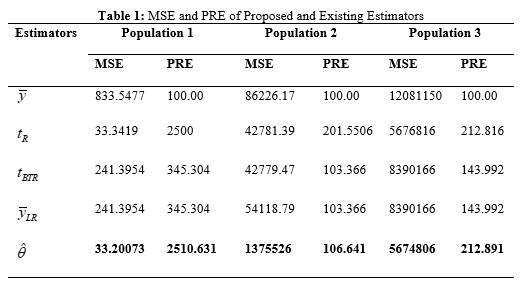# An Efficient Logarithmic Ratio Type Estimator of Finite Population Mean under Simple Random Sampling

## Authors

• Awwal Adejumobi Department of Mathematics, Faculty of Physical Sciences, Kebbi State University of Science and Technology, Aliero, Nigeria https://orcid.org/0000-0003-4048-8576
• Mojeed Abiodun Yunusa Department of Statistics, Faculty of Physical Sciences, Usmanu Danfodiyo University, Sokoto, Nigeria
• Yahqub A. Erinola Department of Mathematics, Faculty of Physical Sciences, Kebbi State University of Science and Technology, Aliero, Nigeria.
• Kabiru Abubakar Department of Statistics, Faculty of Physical Sciences, Usmanu Danfodiyo University, Sokoto, Nigeria

## Keywords:

Auxiliary variable, Logarithmic ratio estimator, Bias, MSE, Efficiency

## Abstract

The use of auxiliary information has become indispensable for improving the exact of estimators of population parameters like the mean and variance of the variable under study. A great variety of the techniques such as the ratio, product, and regression methods of estimation are commonly known in this esteem. In this paper, we propose an efficient logarithmic ratio type estimator for finite population mean estimation under simple random sampling. The expression for the bias and mean squared error (MSE) of the proposed estimator is obtained up to the first order of approximation. The conditions under which the proposed estimator is more efficient than the existing ones are established. An empirical study using three data sets is also conducted to validate the theoretical findings and the results revealed that the suggested estimator is better than the existing estimators considered in the study.

## References

W. G. Cochran, “The Estimation of Yields of the cereal Experiments by Sampling for the Ratio of Grain to Total Produce,” The Journal of Agric. Science, vol. 30, pp. 262-275, 1940.

S. K. Srivastava, “An estimator using auxiliary information in sample survey,” Calcutta Statistical Association

Bulletin, vol. 16, pp. 121-132, 1967.

B. S. V. Sisodia and V. K. Dwivedi, “A modified ratio estimator using coefficient of variation of auxiliary variable,” Journal of India Society of Agricultural Statistics, vol. 33, pp. 13-18, 1981.

S. Bahl and R. K. Tuteja, “Ratio and Product Type Exponential Estimators,” information and Optimization Sciences, vol. 1, pp. 159-163, 1991.

H. P. Singh and R. Tailor, “Use of known Correlation Coefficient in estimating the finite Population mean,” Statistics in Transition, vol. 6, pp. 555-560, 2003.

H. P. Singh, et al. “An improved estimation of Population means using power transformation,” Journal of the Indian Society of Agricultural Statistics, vol. 2, pp. 223-230, 2004.

C. Kadilar and H. Cingi, “Ratio Estimators in Simple random Sampling,” Applied Mathematics and Computation, Vol. 151, pp. 893-902, 2004.

H. P. Singh and R. Tailor, “Estimation of Finite population mean with known Coefficient of variation of an auxiliary character,” Statistica, vol. 3, pp. 301-313, 2005.

R. Singh, et al., “Ratio estimators in simple random sampling using information on auxiliary attribute,” Pakistan Journal of Statistical Operation Research, vol. 1, pp. 47-53, 2008.

H. P. Singh., and R. S. Solanki, “An efficient class of estimators for the population mean using auxiliary information in systematic sampling,” Journal of Statistical Theory and Practice, vol. 2, pp. 274-285, 2012.

S. S. Brar and J. Kaur, “Effect of change of origin of variables on ratio estimator of grain to Total,” The Journal of Agricultural Science, vol. 30, pp. 262-275, 2016.

S. S. Brar, et al., “Some new functional forms of the ratio and product estimators of the population mean.” Revista Investigation Operational, Vol. 41, pp. 416-424, 2020.

J. O. Muili, et al., “Modified ratio-cum-product estimators of population mean using two auxiliary variables,” Asian Journal Researchin Computer Science, vol. 6, pp. 55-65, Article no. AIRCOS.59248, ISSN:25818260, 2020.

A. Audu and R. V. K. Singh, “Exponential-type regressum Compromised imputation Class of estimators,” Journal of Statistics and Management System, 1-15, DOI; 10.1080/09720510/2020.1814501, 2021.

M. A. Yunusa, et al., “An efficient exponential type estimators for estimating finite population mean under simple random sampling,” Annals Computer Science Series, Vol. 19, pp. 46-51, 2021.

A. Audu, et al., “Exponential- Ratio-Type Imputation Class of Estimators using Non conventional Robust Measures of Dispersions,” Asian Journal of Probability and Statistics, vol. 15, pp. 59-74, 2021.

D. Singh and F. S. Chaudhary, “Theory and Analysis of Sample Survey,” New Age International Publisher, 1986.

M. N. Murthy, “Sampling Theory and Methods,” 1967.

A. Adejumobi, et al., “Improved Modified Classes of Regression Type Estimators of Finite Population Mean in the Presence of Auxiliary Attribute,” Oriental Journal of Physical Sciences, vol. 07, pp. 41- 47, 2022.

M. P. Singh, “On the estimation of ratio and product of the population parameters,” Sankhya B., Vol. 7, pp. 231-328, 1965.

M. P. Singh, “Ratio cum product method of estimation,” Metrika, vol. 2, pp. 34–42, 1967.

M. Khoshnevisan, et al., “A general family of estimators for estimating population mean using known value of some population parameter(s),” Far East Journal of Theoretical Statistics, vol. 2, pp. 181– 191, 2007.

P. F. Perri, “Improved ratio-cum-product type estimators,” Statistics in Transition, vol. 2, pp. 51-69, 2007.

L. N. Upadhyaya, et al., “Improved ratio and product exponential type estimator,” Journal of Statistical theory and practice, vol. 5, pp. 285-302, 2011.

F. B. Adebola, et al., “A Class of Regression estimator with cum-Dual-ratio estimators intercept,” International Journal of Statistics and Probability, vol. 4, pp. 42-50, 2015.

R. Singh and M. Kumar, “A note on transformations on auxiliary variable in survey sampling,” MASA, vol. 1, pp. 17-19, 2011.

A. Adejumobi and M. A. Yunusa, “Some Improved Class of Ratio Estimators for Finite Population Variance with the Use of Known Parameters,” LC International Journal of Stem, vol. 3(3), pp. 2708- 7123, September, 2022.

M. A. Yunusa, A. Audu and A. Adejumobi, “Logarithmic Product-Cum-Ratio Type Estimator for Estimating Finite Population Coefficient of Variation,” Oriental Journal of Physical Sciences, vol. 7(2), pp. 82-87, 2022.2023-05-24

## How to Cite


A. Adejumobi, M. . Abiodun Yunusa, Y. . A. Erinola, and K. . Abubakar, “An Efficient Logarithmic Ratio Type Estimator of Finite Population Mean under Simple Random Sampling”, International Journal of Engineering and Applied Physics, vol. 3, no. 2, pp. 700–705, May 2023.

Articles